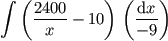# Thread: INTEGRATION OF VARYING ACCELERATION

1. Will someone integrate the equation shown below? You will make a 68 year old man happy.

(2400/120-9t) - 10

t should come out as the instantaneous speed at any given time.

Thanks,
RENATO2.

3. Hint:

∫*(1*∕*t)*dt = ln|t| + C4. Jane,

I know the hint you gave me that 1/t=ln x +c

But I am still having problem getting to the end of integration.

Would you kindly help this old man and fully solve the integration?

Thanks a million.

RENATO5. Okay, how would you integrate 1*∕*(120−9t)?

Well, you know how to do ∫*(1*∕*x)*dx.

So try the substitution x = 120−9t.

Itâs not that tough, is it?6. Jane,

Please see if what I did with substitution is correct:

(2400/120-9t) - 10

let x = 120-9t
dx/dt = -9
dt = dx/-9

{(2400/x) - 10} (dx/-9)

(2400/x)dx/-9 - 10 (dx/-9) or should it be

(2400/x)dx/-9 - 10 ????

Am I on the right track?

RENATO7. Youâre going a bit too far there.

When you get to ∫*(2400*∕*x − 10)*dx*∕*(−9), you should be able to integrate it already.

And donât forget the constant of integration.8. Jane,

How did you arrive with the denominator (x-10)?9.10. See if I got it right. My final answer is

= {(2400/-9) (ln(120-9t)} + {10/9}t

Please show me your answer so I can compare to mine and also learn where I erred.11. Almost correct. First, you forgot the constant of integration. Second, you need to replace x with t.

After integration, you got (2400*⁄*−9)*ln|x| + (10*⁄*9)*x.

ln|x| = ln|120−9t|

and

(10*⁄*9)*x = (1200*⁄*9)−10t (where the (1200*⁄*9) can be absorbed into the constant of integration).

v = −(2400*⁄*9)Â*ln|120−9t| − 10t + v<sub>0</sub>

(where v<sub>0</sub> is your constant of integration).12. Miss Jane,

Now, I can sleep well, knowing that I finally understood the solution to the problem.

I can't thank you enough for your help.

For your help to me, there is a flower blooming in heaven for you.

RENATO13. Miss Jane,

I know that Differential Calculus applications are for finding the rate of change, maxima and minima, etc. Integral, on the other hand, is commonly used in determining areas, volume, work, etc.

My question is, could applications mentioned for diffrential be solved by integral calculus and vice versa? Could any problem be solved by both differential and integral?

Thanks!

RENATO14. Differentiation is normally used for finding rates of change and solving maxima/minima problems, while integration is used for finding areas under curves and volumes of solids of revolution. The two are normally separate. You normally donât do integration to find rates of change, or differentiation to find areas and volumes.

However, differentiation and integration are linked by the fundamental theorem of calculus â and in some areas (e.g. differential equations) you do both. So, depending on your problem, you may have to do both differentiation and integration, or just one or the other.15. THANKS AGAIN!

RENATO16. Miss Jane,

In one problem that I was trying to solve, I used the U substitution. In this case u = 1+x4 or 1+ x^4. In the process of integration I ended with
the equation containing the expression 1/U^1/3. Someone told me that it is a common mistake to integrate it as ln U^1/3.

If he is correct, how about if U = 120 -9t, instead of U = 1 + x^4 ?

THANKS AGAIN.

RENATO17. Sorry, Iâm not sure what you are trying to do. What is the expression youâre trying to integrate?18.Originally Posted by JaneBennet
Almost correct. First, you forgot the constant of integration. Second, you need to replace x with t.

After integration, you got (2400Â*⁄Â*−9)Â*ln|x| + (10Â*⁄Â*9)Â*x.

ln|x| = ln|120−9t|

and

(10Â*⁄Â*9)Â*x = (1200Â*⁄Â*9)−10t (where the (1200Â*⁄Â*9) can be absorbed into the constant of integration).

v = −(2400Â*⁄Â*9)Â*ln|120−9t| − 10t + v<sub>0</sub>

(where v<sub>0</sub> is your constant of integration).

You gave me the answer shown above and I just want to make sure that integration of 1/x = ln x is correct.What if substitution is x=(120 -9t)^2 can we still integate it as ln x?

RENATO